# Fluid Properties and Manometry Civil Engineering (CE) Notes | EduRev

## Civil Engineering (CE) : Fluid Properties and Manometry Civil Engineering (CE) Notes | EduRev

The document Fluid Properties and Manometry Civil Engineering (CE) Notes | EduRev is a part of the Civil Engineering (CE) Course Topic wise GATE Past Year Papers for Civil Engineering.
All you need of Civil Engineering (CE) at this link: Civil Engineering (CE)

Question 1. A closed tank contains 0.5 m thick layer of mercury (specific gravity = 13.6) at the bottom. A 2.0 m thick layer of water lies above the mercury layer. A 3.0 m thick layer of oil (specific gravity = 0.6) lies above the water layer. The space above the oil layer contains air under pressure. The gauge pressure at the bottom of the tank is 196.2 kN/m2. The density of water is 1000 kg/m3 and the acceleration due to gravity is 9.81 m/s2. The value of pressure in the air space is    [2018 : 2 Marks, Set-I]
(a) 92.214 kN/m2
(b) 95.644 kN/m2
(c) 98.922 kN/m2
(d) 99.321 kN/m2
(a)
Solution: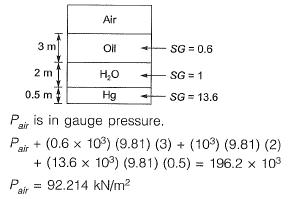Note: It is a closed chamber, hence concept of absolute pressure cannot be applied.
Calculations have to be done in the form of gauge pressure.

Question 2. The figure shows a U-tube having a 5 mm x 5 mm square cross-section filled with mercury (specific gravity = 13.6) up to a height of 20 cm in each limb (open to the atmosphere).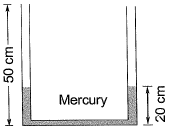If 5 cm3 of water is added to the right limb, the new height (in cm, up to two decimal places) of mercury in the Left limb will b e __________ .      [2017 : 2 Marks, Set-II]
Solution:
Volume of water added = 5 cm3
Cross-section of U tube
= 5 mm x 5 mm = 0.25 cm2
Height of water column in U tube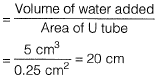Now due to conservation of volume, rise in left limb will be equal to fall in right limb.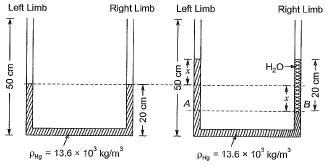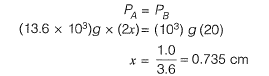So, the new height (in cm to two decimal place of mercury in the left limb will be)
= 20 + 0.74 = 20.74 cm

Question 3. Group I contains the types of fluids while Group II contains the shear stress-rate of shear relationship of different types of fluids, as shown in the figure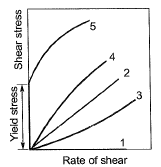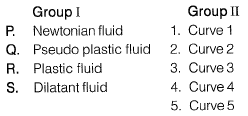The correct match between Group I and Group II is    [2016 : 1 Mark, Set-II]
(a) P-2, Q-4, R-1, S-5
(b) P-2, Q-5, R-4, S-1
(c) P-2, Q-4, R-5, S-3
(d) P-2, Q-1.R-3, S-4
(c)
Solution: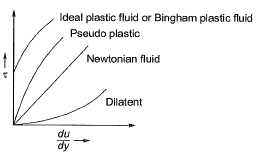Question 4. The dimension for kinematic viscosity is   [2014 : 1 Mark, Set-I]
(a)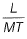(b)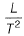(c)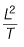(d)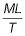(c)
Solution:
The SI unit of kinematic viscosity is m2/s
∴ Dimension of kinematic viscosity [v]=L2/T.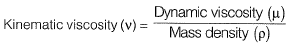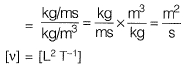Offer running on EduRev: Apply code STAYHOME200 to get INR 200 off on our premium plan EduRev Infinity!

69 docs

,

,

,

,

,

,

,

,

,

,

,

,

,

,

,

,

,

,

,

,

,

;